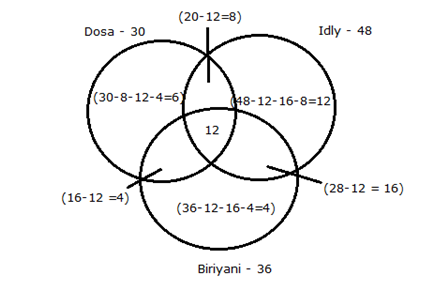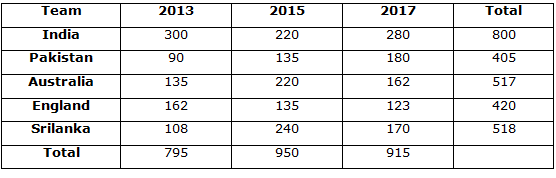# Canara Bank PO Mains 2018 – Quantitative Aptitude Questions Day-45

Dear Readers, Bank Exam Race for the Year 2018 is already started, To enrich your preparation here we have providing new series of Practice Questions on Quantitative Aptitude – Section. Candidates those who are preparing for Canara Bank PO Mains 2018 Exams can practice these questions daily and make your preparation effective.

[WpProQuiz 4501]

1) Arun deposited 25% of his amount in a bank for 5 years which offers simple interest at 15% and 12.5% of his amount in another bank for 3 years which offers SI at 12%. And also 37.5% of his amount lent to his friend for 2 years which offers SI at 8% and rest of the amount deposited for bank for four years which offers SI at 10%. If he got a total interest of Rs.39250, then what was the total amount with Arun?

a) 1lakh

b) 2lakh

c) 1.5lakh

d) 1.8lakh

e) None of these

2) In a river the ratio of the speed of stream and speed of a boat X in still water is 4:7. Again in the same river ratio of the speed of stream and speed of another boat Y in still water is 4:5. What is the ratio of the speed of the first boat X to the speed of the boat Y in still water?

a) 6:5

b) 7:5

c) 4:3

d) 3:2

e) None of these

3) There are two alloys of gold, silver and copper. The first alloy is contains three-fifth of the copper and second alloy contain two-fifth of the silver. The percentage of gold is same in both alloys which is 25%. If the alloyed having 180kg of first alloy and 200kg of the second alloy, how many kg of copper in the alloy?

a) 160 kg

b) 178 kg

c) 184 kg

d) 120 kg

e) None of these

4) If amount of Rs.2800 is obtained after lending out Rs.x at 10% per annum for 4 years as simple interest and amount of Rs.4800 is obtained after lending out Rs.y at 15% per annum for four years as simple interest. Find the value of x and y?

a) 1000, 2000

b) 2000, 3000

c) 1500, 2500

d) 1800, 2800

e) None of these

5) In certain office 30 workers prefer Dosa, 48 workers prefer Idly and 36 workers prefer Briyani. 20 workers prefer Dosa and Idly, 28 workers prefer Idly and Briyani and 16 workers prefer Dosa and Briyani. 12 workers prefer all the items. Then the number of workers who is prefers only one of the items?

a) 70

b) 22

c) 65

d) 64

e) None of these

Direction (6-10): Read the following information carefully and answer the given questions:

Number of runs scored by India in 2017 T20 world cup is 280. Number of runs scored by India in 2015 T20 world cup and 2013 T20 word cup were 60 less and 20 more than the number of runs scored by it in 2017 T20 world cup respectively. Pakistan scored 180 runs in 2017 T20 world cup. Ratio of the number of runs scored by Pakistan in T20 world cups 2017, 2015 and 2013 is 4:3:2. Total number of runs scored by England in 2017, 2015 and 2013 is 420. Total number of runs scored by England in 2015 is equal to the total number of runs scored by Pakistan in 2015 T20 world cup.  Total number of runs scored by England in 2013 T20 world cup was six-fifth of the total number of runs scored by it in 2015 T20 world cup. Number of runs scored by Australia in T20 world cups in 2017 is equal to the total number of runs scored by England in 2013 T20 world cup. Total number of runs scored by Australia in 2017 T20 world cup is 20% more than the total number of runs scored by it in 2013 T20 world cup. Australia scored the run in 2015 T20 world cup is 220. Total number of runs scored by Srilanka in world cups 2017 and 2015 is 170 and 240 respectively. Srilanka scored the runs in 2013 T20 world cup is 20% less than the total number of scored by Pakistan in 2015 world cup.

6) Total number of runs scored by Australia in world cups 2017, 2015 and 2013 together is approximately what percent of the total runs scored by Pakistan in world cup 2015 and 2013 together?

a) 200%

b) 258%

c) 230%

d) 238%

e) 242%

7) In 2018 world cup, India scored the run is 55% more than that of it scored the run in 2015 world cup and England scored the run in 2018 is 140 run more than the number of runs scored by it in 2017 world cup. What is the difference between average number of runs scored by India and England in 2018, 2017, 2015 and 2013 world cup together?

a) 114.5

b) 115.8

c) 117.5

d) 111.5

e) None of these

8) What is the ratio of total number of runs scored in 2013 world cup by all the five teams together to number of runs scored by Pakistan and Australia all the three world cup together?

a) 795:922

b) 825:934

c) 685:824

d) 595:726

e) None of these

9) What is the approximate average number of runs scored in all three world cup scoring the second highest number of runs?

a) 190

b) 182

c) 187

d) 185

e) 196

10) Total number of runs scored by Srilanka and India in all the world cup together is approximately what percent of the runs scored by all the teams in 2015?

a) 128%

b) 139%

c) 148%

d) 158%

e) 162%

Total amount=100x

(100x*25/100*5*15/100)+(100x*12.5/100*3*12/100)+(100x*37.5/100*2*8/100)+(25x*4*10/100)=39250

25x*5*15+12.5x*3*12+37.5x*2*8+25x*4*10=3925000

1875x+450x+600x+1000x=3925000

3925x=3925000

X=1000

Total amount=1000*100=100000

Speed of stream=4x

Speed of boat X=7x

Speed of stream=4y

Speed of boat Y=5y

Speed of stream is same 4x=4y

x=y

Speed of boat X to Y=7x:5y

=7x:5x

=7:5

Silver in second alloy=200*2/5=80

Gold in second alloy=200*1/4=50

Copper in second alloy=200-80-50=70

Copper in first alloy=180*3/5=108

Total copper=108+70=178 kg

2800=x+(x*10*4/100)

280000=140x

x=2000

4800=y+(y*15*4/100)

480000=160y

Y=3000Number of workers prefer only one item= 6+12+4 = 22

Directions (6-10):

India:

Number of runs scored by 2017=280

2015 =280-60=220

2013=280+20=300

Pakistan:

2017=180

2015=180*3/4=135

2013=180*2/4=90

England:

Total number of runs scored by England in 2017, 2015 and 2013 is 420.

2015=135

2013=6/5*135=162

2017=420-135-162=123

Australia:

2017=162

2013=162*100/120=135

2015=220

Srilanka:

2017=170

2015=240

2013=135*80/100=108Required percentage=517*100/225=230%

India 2018=220*155/100=341

Average=(300+220+280+341)/4=1141/4=285.25

England 2018=123+140=263

Average=(162+135+123+263)/4=170.75

Difference=285.25-170.75=114.5

Total scored by all the team in 2013=795

Total scored by Pakistan in all three world cup=405

Total scored by Australia in all three world cup=517

Pakistan + Australia=405+517=922

Required ratio=795:922

2013 second highest score=162

2015 second highest score=220

2017 second highest score=180

Average=162+220+180/3=187

Total scored in 2015=950

Total runs scored by India and Srilanka=800+518=1318

Required percentage=1318*100/950=139%

### Daily Practice Test Schedule | Good Luck

 Topic Daily Publishing Time Daily News Papers & Editorials 8.00 AM Current Affairs Quiz 9.00 AM Current Affairs Quiz (Hindi) 9.30 AM IBPS Clerk Prelims – Reasoning 10.00 AM IBPS Clerk Prelims – Reasoning (Hindi) 10.30 AM IBPS Clerk Prelims – Quantitative Aptitude 11.00 AM IBPS Clerk Prelims – Quantitative Aptitude (Hindi) 11.30 AM Vocabulary (Based on The Hindu) 12.00 PM IBPS Clerk Prelims – English Language 1.00 PM SSC Practice Questions (Reasoning/Quantitative aptitude) 2.00 PM IBPS Clerk – GK Questions 3.00 PM SSC Practice Questions (English/General Knowledge) 4.00 PM Daily Current Affairs Updates 5.00 PM Canara Bank PO Mains – Reasoning 6.00 PM Canara Bank PO Mains – Quantitative Aptitude 7.00 PM Canara Bank PO Mains – English Language 8.00 PM